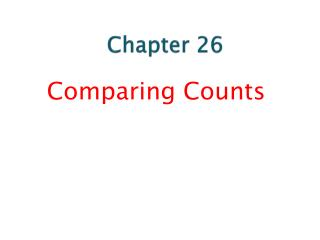DownloadDownload PresentationChapter 26

# Chapter 26

Télécharger la présentation## Chapter 26

- - - - - - - - - - - - - - - - - - - - - - - - - - - E N D - - - - - - - - - - - - - - - - - - - - - - - - - - -
##### Presentation Transcript

1. Chapter 26 Comparing Counts

2. Goodness-of-Fit • A test of whether the distribution of counts in one categorical variable matches the distribution predicted by a model is called a goodness-of-fit test. • Example: A company printed baseball cards. It claimed that 30% of its cards were rookies; 60% veterans and 10% All-Stars. We want to know if their claim is true. Suppose a randomly-selected package of cards has 50 rookies, 45 veterans, and 5 All-Stars. Is this consistent with their claim?

3. Assumptions and Conditions • Counted Data Condition: Check that the data are counts for the categories of a categorical variable. • Independence Assumption: The counts in the cells should be independent of each other. • Randomization Condition: • Sample Size Assumption: We must have enough data for the methods to work. • Expected Cell Frequency Condition: We should expect to see at least 5 individuals in each cell. • This is similar to the condition that np and nq be at least 10 when we tested proportions.

4. Calculations • Since we want to examine how well the observed data reflect what would be expected, it is natural to look at the differences between the observed and expected counts (Obs – Exp). • These differences are actually residuals, so we know that adding all of the differences will result in a sum of 0. That’s not very helpful. • We’ll handle the residuals as we did in regression, by squaring them. • To get an idea of the relative sizes of the differences, we will divide these squared quantities by the expected values.

5. The test statistic, called the chi-square (or chi-squared) statistic, is found by adding up the sum of the squares of the deviations between the observed and expected counts divided by the expected counts: Chi-square statistic

6. Chi-square Model • The chi-square models are actually a family of distributions indexed by degrees of freedom (much like the t-distribution). • The number of degrees of freedom for a goodness-of-fit test is n – 1, where n is the number of categories.

7. Chi-Square P-Values • The chi-square statistic is used only for testing hypotheses, not for constructing confidence intervals. • If the observed counts don’t match the expected, the statistic will be large—it can’t be “too small.” • So the chi-square test is always one-sided. • If the calculated value is large enough, we’ll reject the null hypothesis. • There’s no direction to the rejection of the null model—all we know is that it doesn’t fit.

8. The Chi-Square Calculation • Find the expected values: • Every model gives a hypothesized proportion for each cell. • The expected value is the product of the total number of observations times this proportion. • Compute the residuals:Observed – Expected. • Square the residuals. • Compute the components. Now find the components for each cell.

9. The Chi-Square Calculation (cont.) • Find the sum of the components (that’s the chi-square statistic). • Find the degrees of freedom. It’s equal to the number of cells minus one. • Test the hypothesis. • Use your chi-square statistic to find the P-value. • Large chi-square values mean lots of deviation from the hypothesized model, so they give small P-values.

10. Homework Assignments • Page 712 - 716 • Problem # 3, 5, 7, 9, 11.